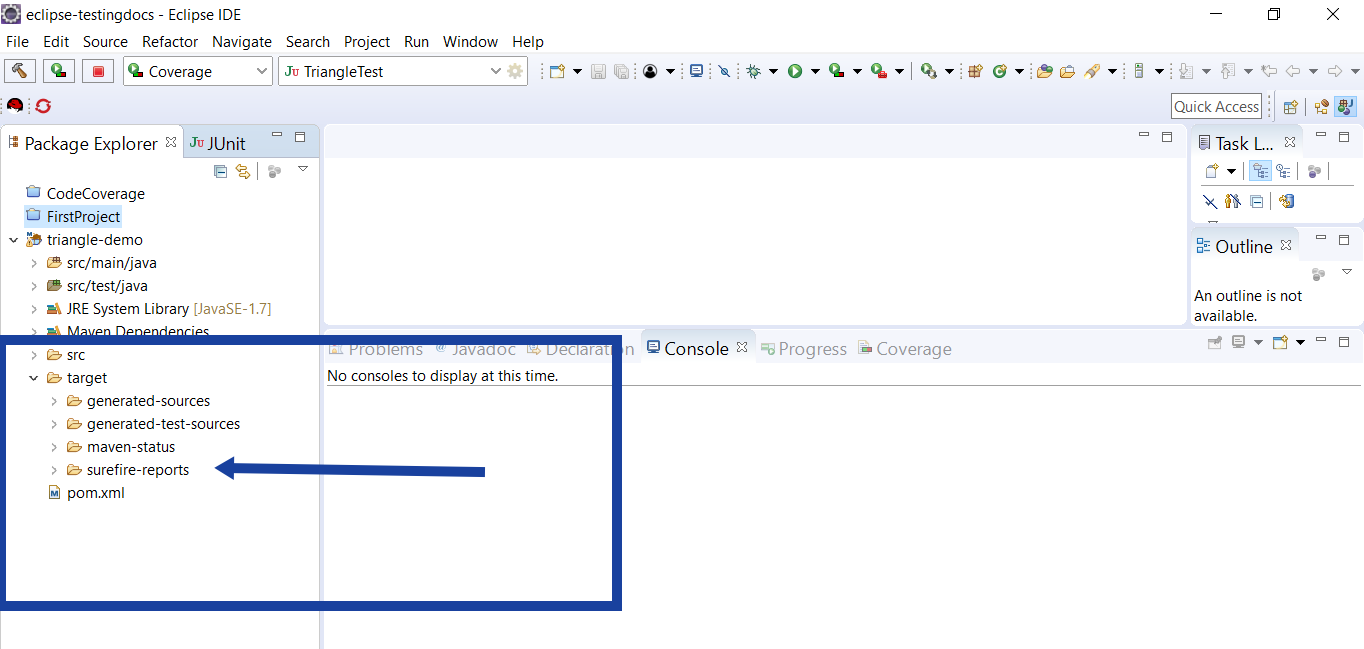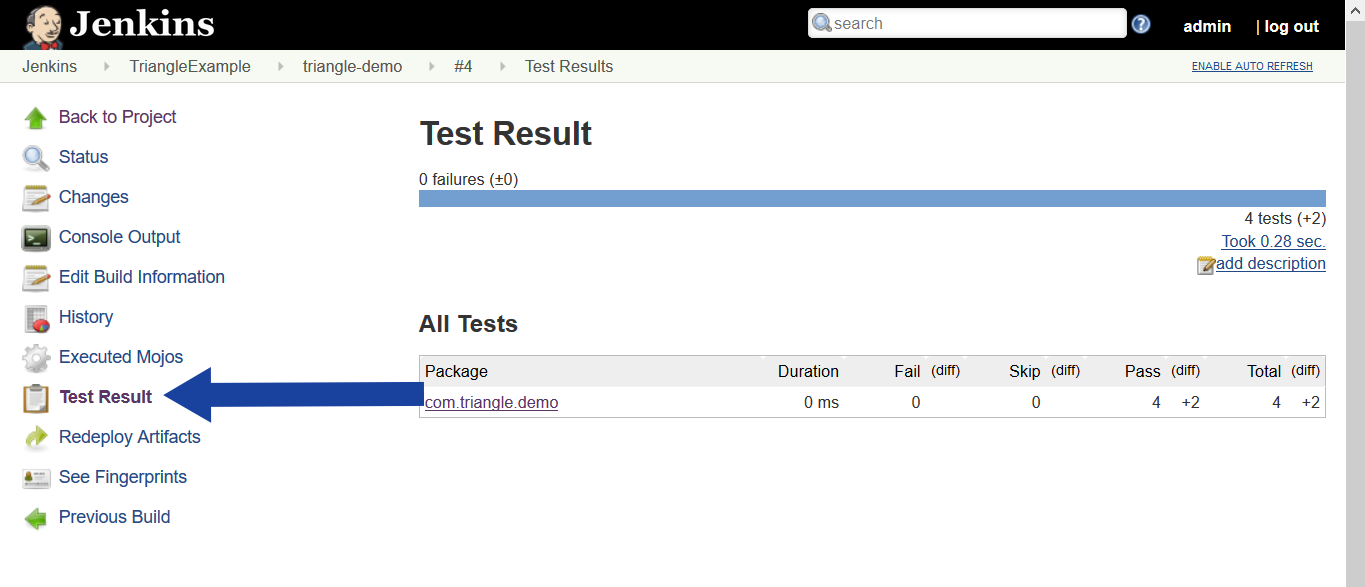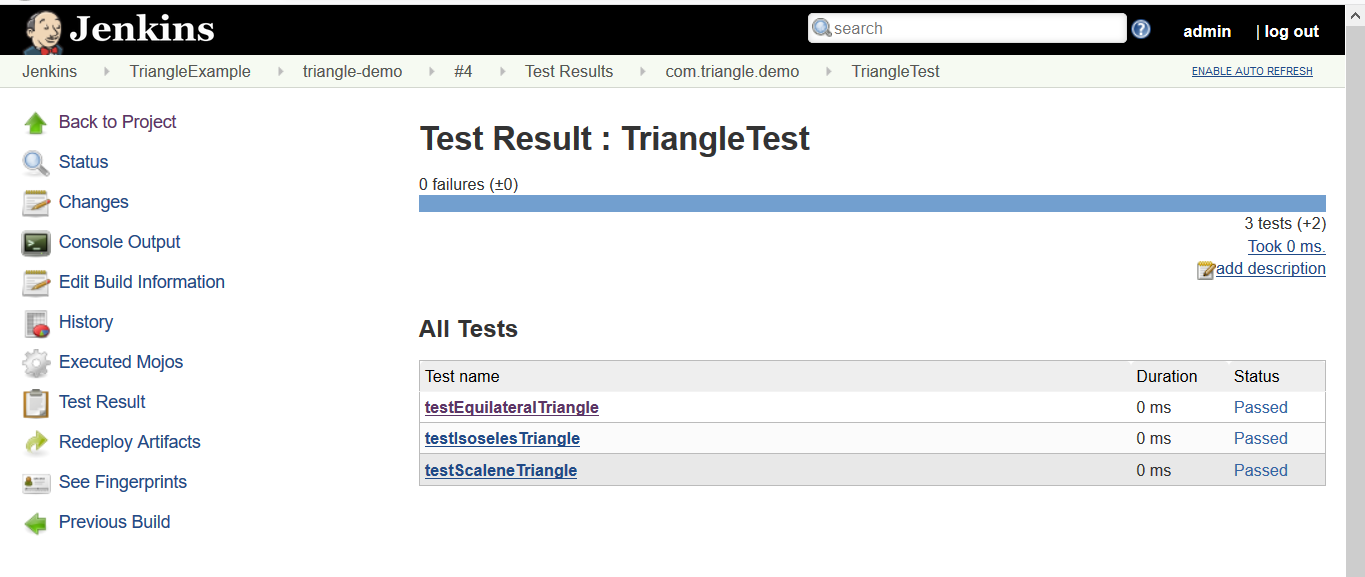# Maven Surefire Plugin Jenkins

Contents

### Introduction

When Maven runs tests in a project, it automatically generates XML based test reports in a directory called surefire-reports in the target under the project directory.### Sample Triangle Tests

```package com.triangle.demo;
public class Triangle { public static final int NOTATRIANGLE = -1; public static final int SCALENE = 0; public static final int ISOSELES = 1; public static final int EQUILATERAL = 2; public static int check(int a, int b, int c) { boolean isTriangle; if((a < b + c) && (b < a + c) && (c < a + b)) isTriangle = true; else isTriangle = false; int triangleType = NOTATRIANGLE; if(isTriangle) { if((a == b) && (b == c)) triangleType = EQUILATERAL; else if((a != b) && (a != c) && (b != c)) triangleType = SCALENE; else triangleType = ISOSELES; } else triangleType = NOTATRIANGLE; return triangleType; }
}```

### Triangle Class Tests

```package com.triangle.demo;
import static org.junit.Assert.*;
import org.junit.Test;
public class TriangleTest { @Test public void testEquilateralTriangle() { assertEquals(Triangle.EQUILATERAL, Triangle.check(7, 7, 7)); } @Test public void testIsoselesTriangle() { assertEquals(Triangle.ISOSELES, Triangle.check(4, 4, 6)); } @Test public void testScaleneTriangle() { assertEquals(Triangle.SCALENE, Triangle.check(6, 7, 9)); }
}```

Let’s add some sample tests to a Maven project and run them using Jenkins build.

### Console Output

Build the project and click on the Console output. You can see that Jenkins reports your tests.

[INFO]
[INFO] — maven-surefire-plugin:2.22.1:test (default-test) @ triangle-demo —

[INFO]
[INFO] ——————————————————-
[INFO] T E S T S
[INFO] ——————————————————-

[INFO] Running com.triangle.demo.AppTest
[INFO] Tests run: 1, Failures: 0, Errors: 0, Skipped: 0, Time elapsed: 0.289 s – in com.triangle.demo.AppTest
[INFO] Running com.triangle.demo.TriangleTest
[INFO] Tests run: 3, Failures: 0, Errors: 0, Skipped: 0, Time elapsed: 0 s – in com.triangle.demo.TriangleTest

[INFO]
[INFO] Results:
[INFO]
[INFO] Tests run: 4, Failures: 0, Errors: 0, Skipped: 0
[INFO]
[JENKINS] Recording test results

————————-

To view the test result, click on the build and Test Report link in the sidebar as shown below.You can click on the package link and drill down the test results of individual tests.